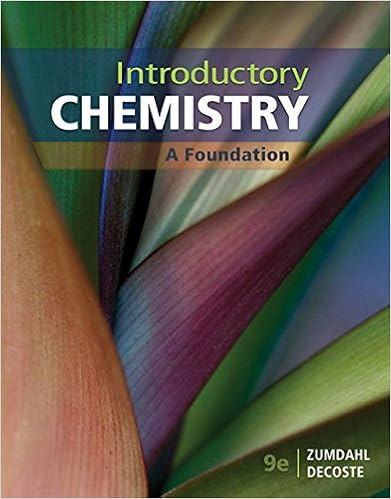# Lab 6 How do buffers work (3) - How do buffers work...

• Lab Report
• 8
• 74% (19) 14 out of 19 people found this document helpful

This preview shows page 1 - 3 out of 8 pages.

##### We have textbook solutions for you!
The document you are viewing contains questions related to this textbook.The document you are viewing contains questions related to this textbook.
Chapter 17 / Exercise 7
Introductory Chemistry: A Foundation
ZumdahlExpert Verified
How do buffers work?Learning Outcomes:Students will apply the Henderson Hasselbalch equation to solve for pH ofbuffer solutions. Students will represent data graphically. Students willmeasure the effects of dilution and acid or base addition to buffer solutionsand interpret the results.Prelab Questions:1.a. What is the pH of a solution formed by combining 50.00 mL of a 0.0855 Macetic acid solution, 25.00 mL of 0.1061 M NaOH solution and 25.00 mL ofdeionized water? Show your work.
b. What is the pH when the solution in 1a is diluted 1 mL in 10 mL total volume?Show your work.
2.What is the pH when 25.00 mL of the solution in #1a has 5.00 mL of a 0.1056 MHCl solution added to it? Show your work.
3.What is the pH when 25.00 mL of the solution in #1a has 5.00 mL of a 0.1056 MNaOH solution added to it? Show your work.
4.What volume of a 0.1048 M NaOH is needed to neutralize (get to theequivalence point) 50.00 mL of a 0.0876 M acetic acid solution? What is the pHat the equivalence point. Show your work.
)
##### We have textbook solutions for you!
The document you are viewing contains questions related to this textbook.The document you are viewing contains questions related to this textbook.
Chapter 17 / Exercise 7
Introductory Chemistry: A Foundation
ZumdahlExpert Verified
5.In the titration of 15.00 mL of a 0.1000 M acetic acid solution by a 0.1000 MNaOH solution, find the pH of the solution being titrated when
=
•••